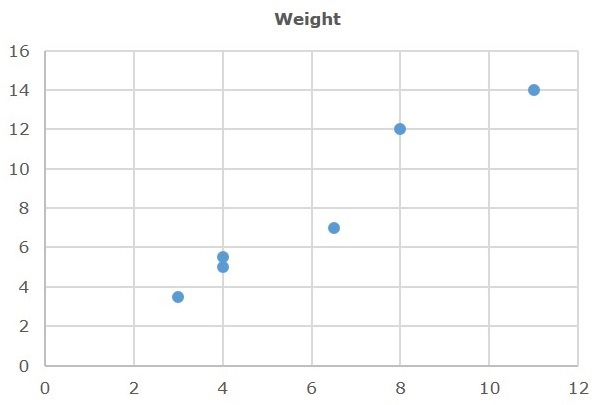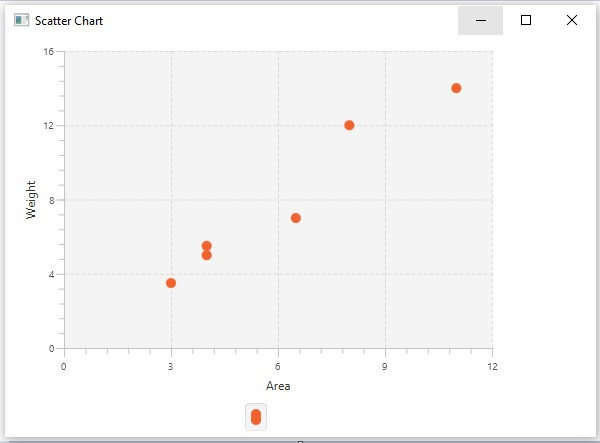# JavaFX - Scatter Chart

A scatterplot is a type of graph which uses values from two variables plotted in a Cartesian plane. It is usually used to find out the relationship between two variables.

Following is a Scatter chart plotted between area and weight.In JavaFX, a Scatter chart is represented by a class named ScatterChart. This class belongs to the package javafx.scene.chart. By instantiating this class, you can create a ScatterChart node in JavaFX.

## Steps to Generate Scatter Chart

To generate an area chart in JavaFX, follow the steps given below.

### Step 1: Creating a Class

Create a Java class and inherit the Application class of the package javafx.application. You can then implement the start() method of this class as follows.

```public class ClassName extends Application {
@Override
public void start(Stage primaryStage) throws Exception {
}
}
```

### Step 2: Defining the Axis

Define the X and Y axis of the area chart and set labels to them. In our example, X axis represents area and the Y axis represents weights.

```//Defining the x axis
NumberAxis xAxis = new NumberAxis(0, 12, 3);
xAxis.setLabel("Area");

//Defining the y axis
NumberAxis yAxis = new NumberAxis(0, 16, 4);
yAxis.setLabel("Weight");
```

### Step 3: Creating the Scatter Chart

Create a line chart by instantiating the class named ScatterChart of the package javafx.scene.chart. To the constructor of this class, pass the objects representing the X and Y axis created in the previous step.

```//Creating the Scatter chart
ScatterChart<String, Number> scatterChart = new ScatterChart(xAxis, yAxis);
```

### Step 4: Preparing the Data

Instantiate the XYChart.Series class and add the data (a series of, x and y coordinates) to the Observable list of this class as follows −

```//Prepare XYChart.Series objects by setting data
XYChart.Series series = new XYChart.Series();
```

### Step 5: Add Data to the Scatter Chart

Add the data series prepared in the previous step to the scatter chart as follows −

```//Setting the data to scatter chart
```

### Step 6: Creating a Group Object

In the start() method, create a group object by instantiating the class named Group. This belongs to the package javafx.scene.

Pass the ScatterChart (node) object created in the previous step as a parameter to the constructor of the Group class. This should be done in order to add it to the group as follows −

```Group root = new Group(scatterChart);
```

### Step 7: Creating a Scene Object

Create a Scene by instantiating the class named Scene, which belongs to the package javafx.scene. To this class, pass the Group object (root) created in the previous step.

In addition to the Root Object, you can also pass two double parameters representing height and width of the screen, along with the object of the Group class as follows.

```Scene scene = new Scene(group ,600, 300);
```

### Step 8: Setting the Title of the Stage

You can set the title to the stage using the setTitle() method of the Stage class. The primaryStage is a Stage object, which is passed to the start method of the scene class as a parameter.

Using the primaryStage object, set the title of the scene as Sample Application as follows.

```primaryStage.setTitle("Sample Application");
```

### Step 9: Adding Scene to the Stage

You can add a Scene object to the stage using the method setScene() of the class named Stage. Add the Scene object prepared in the previous steps using this method as follows.

```primaryStage.setScene(scene);
```

### Step 10: Displaying the Contents of the Stage

Display the contents of the scene using the method named show() of the Stage class as follows.

```primaryStage.show();
```

### Step 11: Launching the Application

Launch the JavaFX application by calling the static method launch() of the Application class from the main method as follows.

```public static void main(String args[]){
launch(args);
}
```

### Example

The following table contains sample data plotted between area and weight.

Area Weight
8 12
4 5.5
11 14
4 5
3 3.5
6.5 7

Following is a Java program which generates a scatter chart depicting the above data using JavaFX.

Save this code in a file with the name ScatterChartExample.java.

```import javafx.application.Application;
import static javafx.application.Application.launch;
import javafx.scene.Group;
import javafx.scene.Scene;
import javafx.stage.Stage;
import javafx.scene.chart.NumberAxis;
import javafx.scene.chart.ScatterChart;
import javafx.scene.chart.XYChart;

public class ScatterChartExample extends Application {
@Override
public void start(Stage stage) {
//Defining the axes
NumberAxis xAxis = new NumberAxis(0, 12, 3);
xAxis.setLabel("Area");

NumberAxis yAxis = new NumberAxis(0, 16, 4);
yAxis.setLabel("Weight");

//Creating the Scatter chart
ScatterChart<String, Number> scatterChart =
new ScatterChart(xAxis, yAxis);

//Prepare XYChart.Series objects by setting data
XYChart.Series series = new XYChart.Series();

//Setting the data to scatter chart

//Creating a Group object
Group root = new Group(scatterChart);

//Creating a scene object
Scene scene = new Scene(root, 600, 400);

//Setting title to the Stage
stage.setTitle("Scatter Chart");

stage.setScene(scene);

//Displaying the contents of the stage
stage.show();
}
public static void main(String args[]){
launch(args);
}
}
```

Compile and execute the saved java file from the command prompt using the following commands.

```javac ScatterChartExample.java
java ScatterChartExample
```

On executing, the above program generates a JavaFX window displaying a scatter chart as shown below.javafx_charts.htm